# 【每日一题20220406】回文数字 #2‍ 回文是指一段数字或者文本，正着读和倒着读都是相同的，例如2002，110011都是回文数字。 请编写一个函数，接收一个数字参数num，判断num中是否包含回文数字，如果包含就返回True否则返回False。个位数字不被视为回文数字。

【示例】

``````def solution(num: int)-> bool:

assert solution(5) is False
assert solution(1215) is True
assert solution(1294) is False
assert solution(141221001) is True
``````
``````def solution(num: int)-> bool:
num_len = len(str(num))
if num_len <= 1:
return False
for i in range(num_len):
for j in range(i+2,num_len+1):
if str(num)[i:j] == str(num)[i:j][::-1]:
return True
return False

assert solution(5) is False
assert solution(1215) is True
assert solution(1294) is False
assert solution(141221001) is True
``````
``````def is_huiwen(num):
while num > 10:
if str(num) == str(num)[::-1]:
return True
num = int(num / 10)
else:
return False

def solution(num: int)-> bool:
return is_huiwen(num) or is_huiwen(int(str(num)[::-1]))
``````
``````def solution(num):
if not isinstance(num, int) or num < 0:
return 'Not valid'

nums = str(num)
for i in range(len(nums)):
for j in range(i+1, len(nums)):
if nums[i: j+1] == nums[i: j+1][::-1]:
return True
return False
``````

``````
def solution(num: int)-> bool:
num= str(num)
if len(num) == 1:
return False
else:
for i in range(len(num)):
for j in range(i+1,len(num)):
if num[i:1+j] == num[i:1+j][::-1]:
return True
return False

``````
``````def solution(num: int)-> bool:
if len(str(num))<=1:
return False
else:

if len([True for i in range(len(str(num)))  for j in range(i+2,len(str(num))+1)  if str(num)[i:j]== str(num)[i:j][::-1]  ])>=1:
return True
else:
return False

assert solution(5) is False
assert solution(1215) is True
assert solution(1294) is False
assert solution(141221001) is True
``````
``````    def solution(num: int) -> bool:
num_s = str(num)
num_len = len(num_s)
if num > 10:
for i in range(0, num_len):
min_len = min(i, num_len - i)
for j in range(0, min_len + 1):
if num_s[i - j - 1:i] == num_s[i:i + j + 1] or num_s[i - j - 1:i] == num_s[i + 1:i + j + 2]:
return True
return False

assert solution(5) is False
assert solution(1215) is True
assert solution(1294) is False
assert solution(141221001) is True
assert solution(11) is True
``````
``````def fun(num: int) -> bool:
nums_str = str(num)
if nums_str == ''.join(reversed(nums_str)) and len(nums_str) > 1:
return True
else:
return False

def solution(num: int) -> bool:
l = len(str(num))
str_num = str(num)
for i in range(l - 1):
for j in range(i + 1, l):
if fun(int(str_num[i:j + 1])):
return True
return False

assert solution(5) is False
assert solution(1215) is True
assert solution(1294) is False
assert solution(141221001) is True

``````

``````def solution(num: int) -> bool:
length = len(str(num))
for i in range(length):
for j in range(length - i):
ele = str(num)[j:j + i + 1]
if len(ele) <= 1:
print(f"个位数字{str(num)[j:j + i + 1]}不被视为回文数字。")
elif ele == ele[::-1]:
return True
return False

assert solution(5) is False
assert solution(1215) is True
assert solution(1294) is False
assert solution(141221001) is True
``````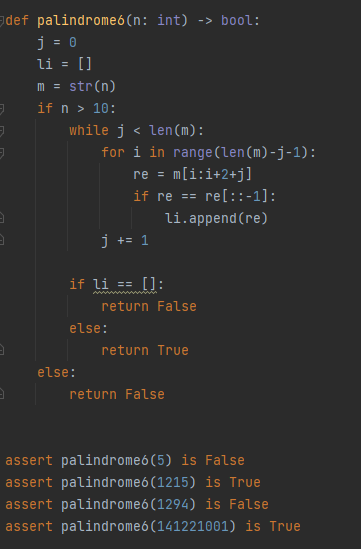``````def solution(num: int)-> bool:
if len(str(num))==1:
return False
for i in range(len(str(num))):
for j in range(i + 2, len(str(num))+1):
if str(num)[i:j] == str(num)[i:j][::-1]:
return True
return False
``````

``````def solution(num: int) -> bool:
if num < 10:
return False

num = str(num)
for n in range(len(num) - 1):
end_index = num[n + 1::].find(num[n])
if end_index != -1 and num[n:end_index + n + 2] == num[n:end_index + n + 2][::-1]:
return True
else:
return False
``````
1 Like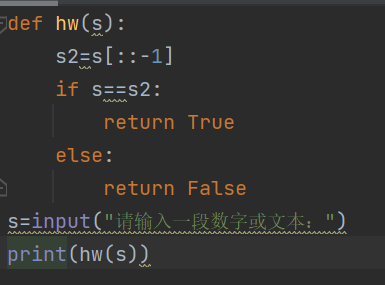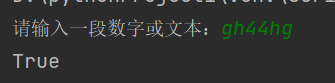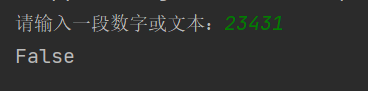``````def solution(num: int) -> bool:
nums = str(num)
if len(nums) < 2:
return False
else:
count = len(nums)
for i in range(0, count-1):
if nums[i : count] == nums[i:count][::-1]:
return True
if nums[::-1][i:count] == nums[::-1][i:count][::-1]:
return True
return False
``````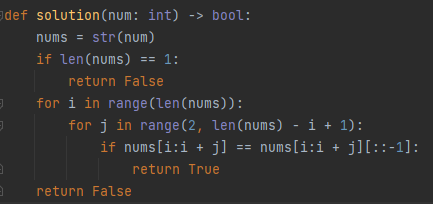``````def solution(num: int)-> bool:
st = str(num)
s = ""
li = []
for i in range(2,len(st)+1):
for j in range(0,len(st)):
s = st[j:j+i]
li = list(s)
li.reverse()
if len(s)>1 and s == "".join(li):
return True
return False

assert solution(5) is False
assert solution(1215) is True
assert solution(1294) is False
assert solution(141221001) is True
assert solution(121) is True
``````